# 2nd PUC Physics Question Bank Chapter 5 Magnetism and Matter

## Karnataka 2nd PUC Physics Question Bank Chapter 5 Magnetism and Matter

### 2nd PUC Physics Magnetism and Matter NCERT Text Book Questions and Answers

Question 1.
Answer the following questions regarding earth’s magnetism:
(a) A vector needs three quantities for its specification. Name the three independent quantities conventionally used to specify the earth’s magnetic field.
(b) The angle of dip at a location in southern India is about 18°. Would you expect a greater or smaller dip angle in Britain?
(c) If you made a map of magnetic field lines at Melbourne in Australia, would the lines seem to go into the ground or come out of the ground?
(d) In which direction would a compass free to move in the vertical plane point to, is located right on the geomagnetic north or south pole?
(e) The earth’s field, it is claimed, roughly approximates the field due to a dipole of magnetic moment 8 x 1022 JT-1 located at its centre. Check the order of magnitude of this number in some way.
(f) Geologists claim that besides the main magnetic N-S poles, there are several local poles on the earth’s surface oriented in different directions. How is such a thing possible at all?
(a) The three quantities are:-

• Magnetic declination
• Angle of dip and
• The horizontal component of the earth’s magnetic field.

(b) Britain is close to magnetic north pole. Therefore, the angle of dip is greater in Britain than in India.  It is about 70°.

(c) The magnetic field lines of the earth could seem to come out of the ground. It is because, Melbourne (Australia) is in Southern hemisphere.

(d) The magnetic compass needle can point in any direction on the geomagnetic north or South pole. It is because the horizontal component of the earth’s field is zero at these points
(∵ The earth’s field is vertical).

(e) The magnetic field at a point on the equatorial line of the magnetic dipole (earth as a magnetic dipole) is given by,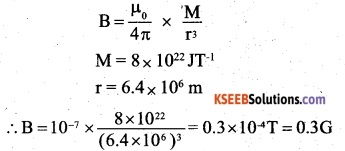It is really of the order of the observed field of earth

(f) The earth’s magnetic field is only approximately a dipole field. The local N>S poles may be oriented due to the different deposits of magnetised minerals.Question 2.
(a) The earth’s magnetic field varies from point to point in space. Does it also change with time? If so, on what time scale does it change appreciably?
(b) The earth’s core is known to contain iron. Yet geologists do not regard this as a source of the earth’s magnetism. Why?
(c) The charged currents in the outer conducting regions of the earth’s core are thought to be responsible for earth’s magnetism. What might be the ‘battery’ (i.e., the source of energy) to sustain these currents?
(d) The earth may have even reversed the direction of its field several times during its history of 4 to 5 billion years. How can geologists know about the earth’s field in such a distant past?
(e) The earth’s field departs from its dipole shape substantially at large distances (greater than about 30,000 km). What agencies may be responsible for this distortion?
(f) Interstellar space has an extremely weak magnetic field of the order of 1012 T. Can such a weak field be of any significant consequence? Explain.
[Note: Exercise 5.2 is meant mainly to arouse your curiosity. Answers to some questions above are tentative or unknown. Brief answers wherever possible are given at the end. For details, you should consult a good text on geomagnetism.]
(a) Yes, it changes with time. After a few hundred years, the earth’s magnetic field undergoes an appreciable change.

(b) The temperature inside the earth is so high that it is impossible for the iron to remain as a magnet and act as a source of magnetic field. The magnetic field due to the earth is considered to be due to the circulating electric currents induced in the iron in the molten state and other conducting materials inside the earth.

(c) A possible explanation can be the phenomenon of radioactivity.

(d) Analysis of the rock magnetism /earth’s magnetic field gets recorded in certain rocks during solidification, (through weekly) provides clues to the geomagnetic history.

(e) At large distances, the earth’s magnetic field gets modified by the fields produced by the motion of ions in the earth’s ionosphere.

(f) A weak field of the order of 10-12 T can cause the charged particles to move along circular paths of very large radii. Over a small distance, we may not be able to notice the deflection in the path of the charged particles but over large interstellar distance, the distance is quite noticeable.

Question 3.
A short bar magnet placed with its axis at 30° with a uniform external magnetic field of 0.25 T experiences a torque of magnitude equal to 4.5 x 10-2 What is the magnitude of magnetic moment of the magnet?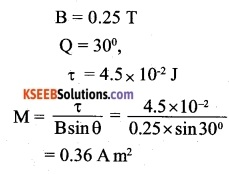Question 4.
A short bar magnet of magnetic moment m = 0.32 JT-1 is placed in a uniform magnetic field of 0.15 T. If the bar is free to rotate in the plane of the field, which orientation would correspond to its
(a) stable, and
(b) unstable equilibrium? What is the potential energy of the magnet in each case?
(a) When is parallel to g, the magnet is in stable equilibrium
U = – MB = -0.32 x 0.15 = – 4.8 x 10-2 J

(b) When $$\vec { M }$$ is antiparallel to g, the magnet is in unstable equilibrium.
U = MB = 0.32 x 0.15
= 4.8 x 10-12 J.

Question 5.
A closely wound solenoid of 800 turns and area of cross-section 2.5 x 10-4 m2 carries a current of 3.0 A. Explain the sense in which the solenoid acts like a bar magnet. What is its associated magnetic moment?
When current is passed through the solenoid, magnetic field is produced along its. axis. The magnetic field lines emanate from its one end and enter the other just as in case of a bar magnet. The two ends of the solenoid act as the two poles of a bar magnet.
Here, the number of turns in the solenoid = 800
I = 3A
A=2.5 x 10-4m2
The magnetic moment of the solenoid,
M = (IA) x number of turns
= 3 x 2.5 x 10-4 x 800
= 0.6 Am2Question 6.
If the solenoid in Exercise 5.5 is free to turn about the vertical direction and a uniform horizontal magnetic field of 0.25 T is applied, what is the magnitude of torque on the solenoid when its axis makes an angle of 30° with the direction of applied field?
Here, τ = M B sin θ
M = 0.6 A m2 B = 0.25 T
θ= 30°
∴ τ = 0.6 x 0.25 x sin 30° = 0.075Nm

Question 7.
A bar magnet of magnetic moment 1.5 J T-1 lies aligned with the direction of a uniform magnetic field of 0.22 T.
(a) What is the amount of work required by an external torque to turn the magnet so as to align its magnetic moment:
(i) normal to the field direction,
(ii) opposite to the field direction?
(b) What is the torque on the magnet in cases (i) and (ii)?
(a) M = 1.5 J-1
B = 0.22 T
The work done to rotate the magnet from the position θ1 to θ2 is given by
MB(cos θ1 – cos θ2)
(i) θ1=0° , θ2 = 90°
∴ W = 1.5 x 0.22 (cos0°-cos 90°)
= 1.5 x 0.22 (1-0)= 1.5 x 0.22
= 0.33 J

(ii) θ1 =0° θ2 = 180°
∴ W = 1.5×0.22 (cos0° – cos 180°)
= 1.5 x 0.22 (1-(-1))
= 1.5 x 0.22×2
= 0.665 J

(b) The torque acting on the magnet is given by
τ= MB sin θ
θ = 90°
τ = 1.5 x 0.22 x sin 90°
=1.5 x 0.22×1
= 0.33 Nm
θ = 180°

Question 8.
A closely wound solenoid of 2000 turns and area of cross-section 1.6 × 10-4 m2carrying a current of 4.0 A, is suspended through its centre allowing it to turn in a horizontal plane.
(a) What is the magnetic moment associated with the solenoid?
(b) What is the force and torque on the solenoid if a uniform horizontal magnetic field of 7.5 x 10-2 T is set up at an angle of 30° with the axis of the solenoid?
Number of turns of the solenoid = 2,000
I = 4A
A= 1.6x 10-4m2

(a) The magnetic moment of the solenoid,
M = (IA) x number of turns
= 4 x 1.6 x 10-4 x 2000
= 1.28 Am2

(b) τ-MB sin θ
B = 7.5 x 10-2T
θ = 30°
τ -1.28 x 7.5 x 10-2 x sin30°
= 0.048 Nm
The net force on the solenoid is zero.Question 9.
A circular coil of 16 turns and radius 10 cm carrying a current of 0.75 A rests with its plane normal to an external field of magnitude 5.0 x 10-2 The coil is free to turn about an axis in its plane perpendicular to the field direction. When the coil is turned slightly and released, it oscillates about its stable equilibrium with a frequency of 2.0 s-1. What is the moment of inertia of the coil about its axis of rotation? ,
a = 10 cm = 0.1 m
n = 16
I = 0.75 A
B = 5 x 10-2T
V = 2 s-1            .
The magnetic moment associated with the coil, M = n l A
nl x πa2
= 16 × 0.75 x n x(0.1)2 = 0.377 Am2
If I is moment of inertia of the coil about its axis of rotation, then its frequency of oscillation is given by,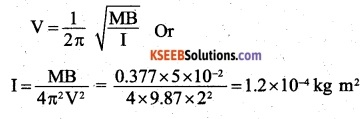Question 10.
A magnetic needle free to rotate in a vertical plane parallel to the magnetic meridian has its north tip pointing down at 22° with the horizontal. The horizontal component of the earth’s magnetic field at the place is known to be 0.35 G. Determine the magnitude of the earth’s magnetic field at the place.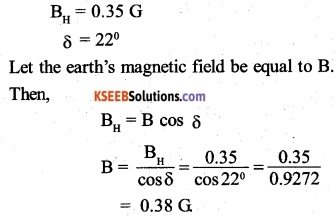Question 11.
At a certain location in Africa, a compass points 12° west of the geographic north. The north tip of the magnetic needle of a dip circle placed in the plane of magnetic meridian points 60° above the horizontal. The horizontal component of the earth’s field is measured to be 0.16 G Specify the direction and magnitude of the earth’s field at the location.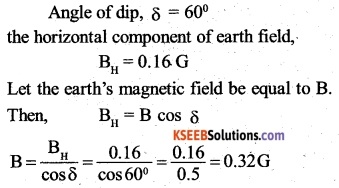Thus, the earth’s magnetic field has a magnitude of 0.32 G and lies in a vertical plane 12° west of the geographic medium making an angle of 60° (upwards) with the horizontal.

Question 12.
A short bar magnet has a magnetic moment of 0,48 J T-1. Give the direction and magnitude of the magnetic field produced by the magnet at a distance of 10 cm from the centre of the magnet on
(a) The axis,
(b) The equatorial lines (normal bisector) of the magnet.
M = 0.48 J T-1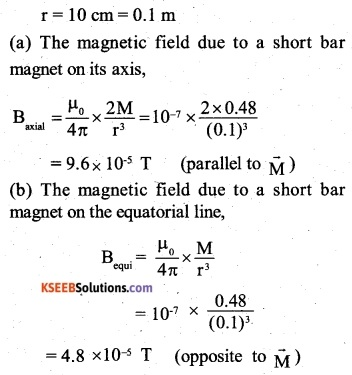Question 13.
A short bar magnet placed in a horizontal plane has its axis aligned along the magnetic north-south direction. Null points are found on the axis of the magnet at 14 cm from the centre of the magnet. The earth’s magnetic field at the place is 0.36 G and the angle of dip is zero. What is the ‘ total magnetic field on the normal bisector of the magnet at the same distance as the null-point (i.e., 14 cm) from the centre of the magnet? (At null points, field due to a magnet is equal and opposite to the horizontal component of earth’s magnetic field.)
In this position of the magnet, the null points lie on its axial line.
At the null point,
Baxial =BH
Let M be the magnetic dipole moment of the magnet, Then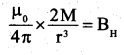At the same distance on the normal bisector (equatorial line) of the magnet, the magnetic field due to the short bar magnet,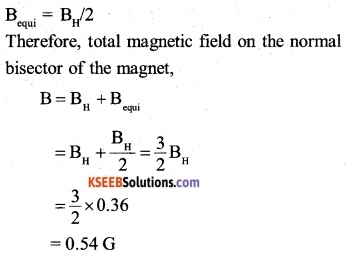Question 14.
If the bar magnet in exercise 5.13 is turned around by 180°, where will the new null points be located?
In this position of the magnet, the null points lie on its equatorial line,
At the null point,
Bequi = BH
Let M be the magnetic dipole moment of the magnet and r. be the distance of the null point from the centre of the magnet.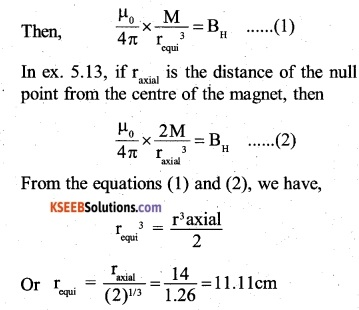Question 15.
A short bar magnet of magnetic moment 5.25 x 10-2 J T is placed with its axis perpendicular to the earth’s field direction. At what distance from the centre of the magnet, the resultant field is inclined at 45° with earth’s field on
(a) its normal bisector and
(b) its axis. Magnitude of the earth’s field at the place is given to be 0.42

G. Ignore the length of the magnet in comparison to the distances involved.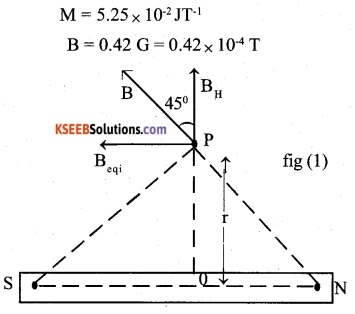(i) On its normal bisector:
Fig (1) shows the point P on the normal bisector of the magnet, where the resultant magnetic field B is inclined at 45°, with the earth’s field.
It can happen only, if Bequi is equal to the horizontal component of the earth’s field (BH)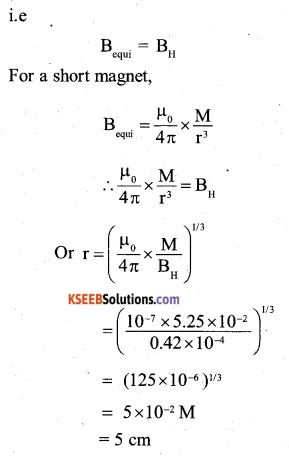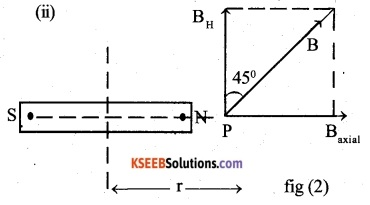Fig (2) shows the point P on the axis of the magnet, where the resultant filed is inclined at 45° with the earth’s magnetic field.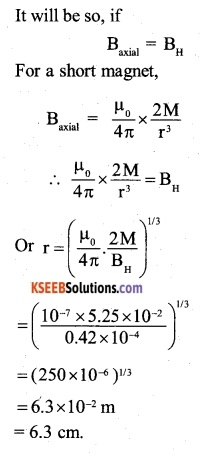### 2nd PUC Physics Magnetism and Matter Additional Exercises

Question 16.
(a) Why does a paramagnetic sample display greater magnetisation (for the same magnetising field) when cooled?
(b) Why is diamagnetism, in contrast, almost independent of temperature?
(c) If a toroid uses bismuth for its core, will the field in the core be (slighty) greater or (slightly) less than when the core is empty?
(d) Is the permeability of a ferromagnetic material independent of the magnetic field? If not, is it more for lower or higher fields?
(e) Magnetic field lines are always nearly normal to the surface of a ferromagnet at every point. (This fact is analogous to the static electric field lines being normal to the surface of a conductor at every point.) Why?
(f) Would the maximum possible magnetisation of a paramagnetic sample be of the same order of magnitude as the magnetisation of a ferromagnet?
(a) When cooled, the tendency of the thermal agitation to disrupt the alignment of magnetic dipoles decreases in case of paramagnetic materials. Hence they display greater magnetisation.

(b) The atoms of a diamagnetic do not have intrinsic magnetic dipole moment. On placing a diamagnetic sample in a magnetic field, the magnetic moment of the sample is always opposite to the direction of the field. It is not affected by the thermal motion of the dipoles.

(c) Since bismuth is diamagnetic, the field in the core coil be sightly less than that when core is empty.

(d) No, the permeability of a ferromagnetic material depends upon the applied magnetic .field. Its value is large for the small values of the applied field. The graph between B and H is of the shape as shown:-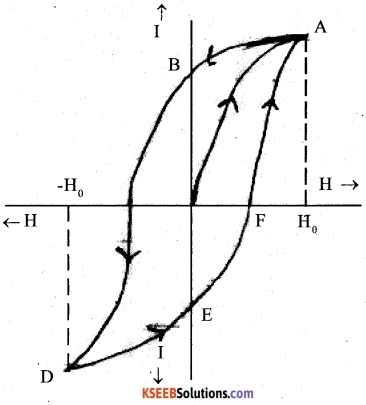It follows that value B is quite large for comparatively small value of H.

(e) One of the reason for the fact is that, when a material has µr > > 1, the field lines meet the material nearly normally.

(f) Yes, a paramagnetic sample with saturated magnetisation will have the same .order of magnetisation as the magnetisation of a ferromagnetic substance. However, the saturated magnetisation will require magnetising field too high to achive. Further, there may be a minor difference in the strengths of the atomic dipoles of paramagnetic and ferromagnetic materials.Question 17.
(a) Explain qualitatively on the basis of domain picture the irreversibility in the magnetisation curve of a ferromagnet.
(b) The hysteresis loop of a soft iron piece has -a much smaller area than that of a carbon steel piece. If the material is to go through repeated cycles of magnetisation, which piece will dissipate greater heat energy?
(c) A system displaying a hysteresis loop such as a ferromagnet, is a device for storing memory?’ Explain the meaning of this statement.
(d) What kind of ferromagnetic material is used for coating magnetic tapes in a cassette player, or for building ‘memory stores’ in a modern computer?
(e) A certain region of space is to be shielded from magnetic fields. Suggest a method.
(a) Refer to the topic of ‘Hysteresis’ on page 195.

• A piece of carbon steel will dissipate a greater amount of heat energy as its hysteresis loop has greater area.
• The magnetisation produced in a ferromagnet does not have a unique value corresponding to the applied magnetising field.

In addition, the magnetisation produced depends on the history of the magnetisation i.e. the number of cycles of magnetisation, it has been taken through. In other words, the value of magnetisation of a ferromagnet is a record or memory of its magnetisation. If information bits can be made corresponding to the cycles of magnetisation, the system displaying the hysteresis loop of the ferromagnet can act as a device for storing the information.

• ceramics are used for coating magnetic tapes in a cassette player or for building memory stores in a modem computer. Ceramics are specially treated barium iron oxides and are also called ferrates.
• The shielding of the region can be done by surrounding it with soft iron rings. The magnetic field lines will be drawn into the rings and the enclosed region will become free of magnetic field.

Question 18.
A long straight horizontal cable carries a current of 2.5 A in the direction 10° south of west to 10“ north of east. The magnetic meridian of the place happens to be 10° west of the geographic meridian. The earth’s magnetic field at the location is 0.33 G, and the angle of dip is zero. Locate the line of neutral points (ignore the thickness of the cable). (At neutral points, magnetic field due to a current-carrying cable is equal and opposite to the horizontal component of earth’s magnetic field.)
The magnetic N-S line is 10° west of geographical N-S line as shown in the figure. The straight horizontal cable x y is 10° north of east.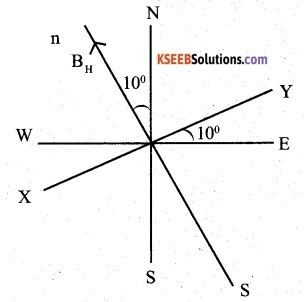Here,
δ=0° and B = 0.33 G
The horizontal component of the earth’s field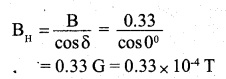= 0.33 G = 0.33 x 10-4 T At a point below the cable, the direction of the magnetic field produced is same as that of BH, while at a point above the cable, neutral point lies above the cable. If the distance of the neutral point from the wire is ‘a’, then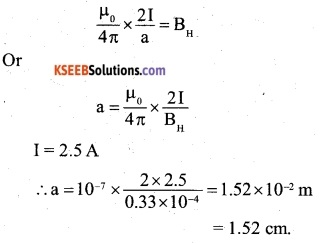Question 19.
A telephone cable at a place has four long straight horizontal wires carrying a current of 1.0 A in the same direction east to west. The earth’s magnetic field at the place is 0.39 G, and the angle of dip is 35°. The magnetic declination is nearly zero. What are the resultant magnetic fields at points 4.0 cm below the cable?
The earth’s magnetic field, B = 0.39 G;
δ=35°
Therefore, horizontal component of the earth’s  magnetic field,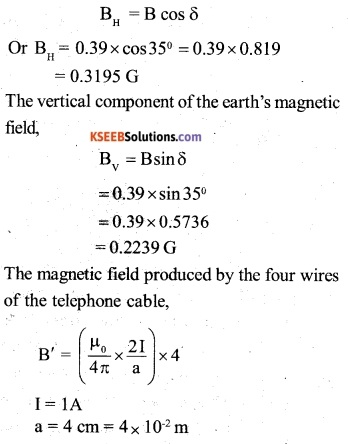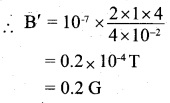Resultant field below the cable:
According to the right hand thumb rule, the direction of b’ at a point below the cable will be opposite to BH.
Therefore, at a point 4 cm above the cable,
B’H = BH + B’
0.3195 + 0.2
= 0.5195 G
Bv = 0.2237 G
Therefore, the resultant magnetic field above the cable,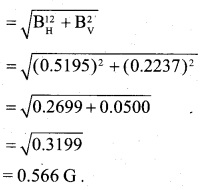Question 20.
A compass needle free to turn in a horizontal plane is placed at the centre of circular coil of 30 turns and radius 12 cm. The coil is in a vertical plane making an angle of 45° with the magnetic meridian. When the current in the coil is 0.35 A, the needle points west to east.
(A) Determine the horizontal component of the earth’s magnetic field at the location.
(B) The current in the coil is reversed, and the coil is rotated about its vertical axis by an angle of 90° in the anti clockwise sense looking from above. Predict the direction of the needle. Take the magnetic declination at the places to be zero.
(a) The horizontal component of the earth’s magnetic field (BH) acts along the magnetic N – S line. As the coil is placed in a vertical plane making angle 45° with the magnetic meridian, the magnetic field B produced by the coil on passing current through it, will be along normal to the plane of the coil, in a direction making angle of 45° with the W-E line. The compass needle placed at the centre of the coil points west to east.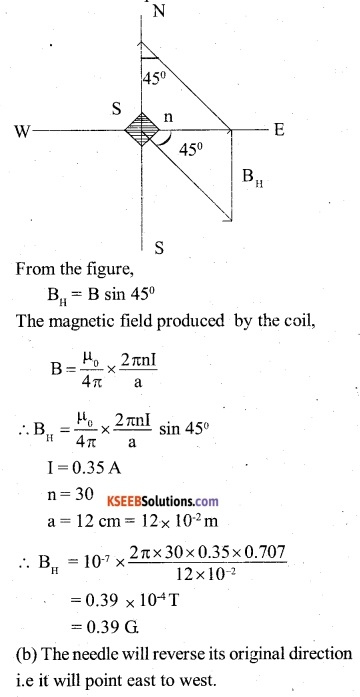Question 21.
A magnetic dipole is under the influence of two magnetic fields. The angle between the field directions is 60°, and one of the fields has a magnitude of 1.2 x 10-2 If the dipole comes to stable equilibrium at an angle of 15° with this field, what is the magnitude of the other field?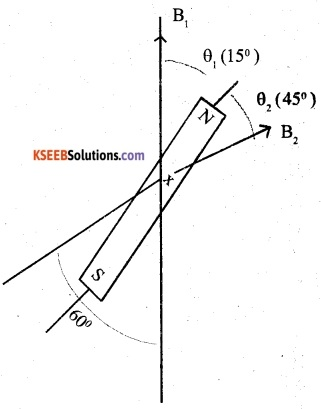B1= 1.2 × 10-2 T
Inclination of the dipole with Bl, θ, =15°
Therefore, inclination of the dipole with B2
θ2= 60-15 = 45°
As the dipole is in equilibrium; the torque on the dipole due to the filed B1: is equal and opposite to that due to B2 . If M is the magnetic dipole moment of the dipole, then,
MB1 sinθ1=MB2 sinθ2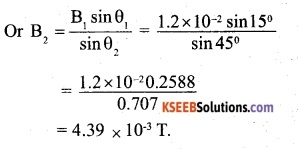Question 22.
A monoenergetic (18 keV) electron beam initially in the horizontal direction is subjected to a horizontal magnetic field of . 0.04G normal to the initial direction. Estimate the up or down deflection of the beam over a distance of 30 cm (me = 9.11 x 10-31 kg). [Note: Data in this exercise are so chosen that the answer will give you ah Idea of the effect of earth’s magnetic field on the motion of the electron beam from the electron gun to the screen in a TV set.]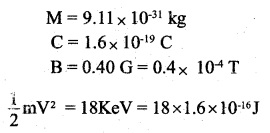suppose that the electron moves along a circular path of radius r under the effect of magnetic field. Then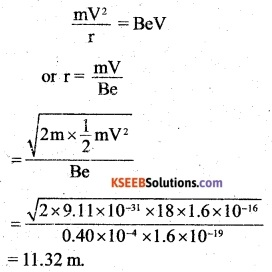Figure shows an electron covering a circular path PQ C = 30 cm = 0.3 m and of radius OP = OQ= 11.32m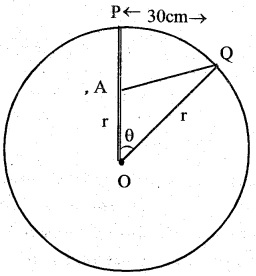It follows the electron will undergo a deflection PA in downward direction, while it covers a distance PQ. IF θ is angle subtended by PQ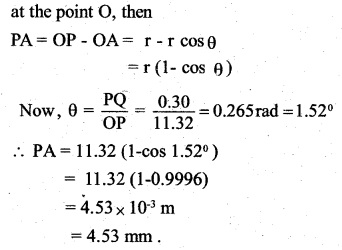Question 23.
A sample of paramagnetic salt contains 2.0 x 1024 atomic dipoles each of dipole moment 1.5 x 10-23 JT-1. The sample is placed under a homogeneous magnetic field of 0.64 T, and cooled to a temperature of 4.2 K. The degree of magnetic saturation achieved is equal to 15%. What is the total dipole moment of the sample for a magnetic field of 0.98 T and a temperature of 2.8 K? (Assume Curie’s law)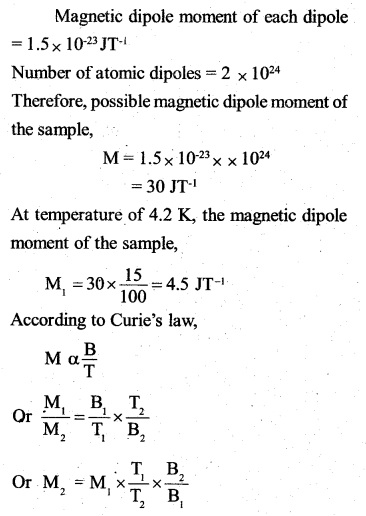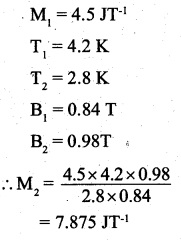Question 24.
A Rowland ring of mean radius 15 cm has 3500 turns of wire wound on a ferromagnetic core of relative permeability 800. What is the magnetic field B in the core for a magnetising current of 1.2 A?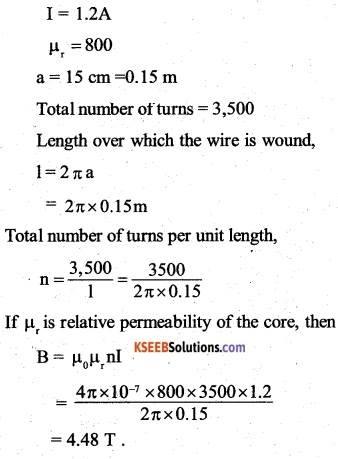Question 25.
The magnetic moment vectors µs and µ, associated with the intrinsic spin angular momentum S and orbital angular momentum 1, respectively, of an electron are predicted by quantum theory (and verified experimentally to a high accuracy) to be given by:
µs=-(e/m)S,
µ1=- (e/ 2m) 1,
Which of these relations is in accordance with the result expected classically? Outline the derivation of the classical result.
The relation $$\vec { { \mu }_{ 1 } } =(-e/2m)\vec { L }$$
is in accordance with the classical physics. It can be derived classical as below:
An electron revolving in an orbit of radius r is associated with magnetic moment.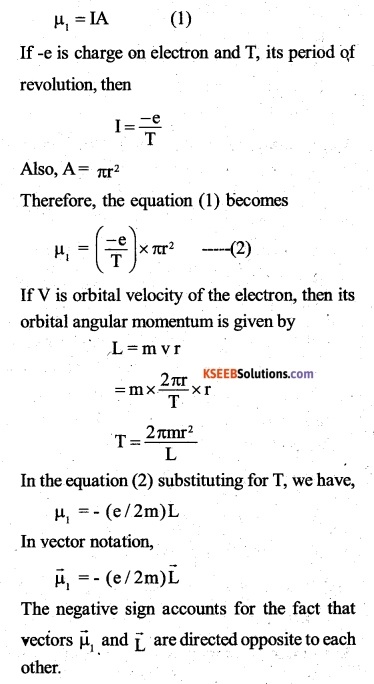### 2nd PUC Physics Magnetism and Matter Competitive Exam Questions and Answers

Question 1.
The magnetic lines of force inside a bar magnet:
(A) Are from north-pole to south-pole of the magnet
(B) Do not exist
(C) Depend upon the area of cross-section of bar magnet
(D) Are from south-pole to north-pole of magnet
(D) Are from south-pole to north-pole of magnet

Question 2.
A magnetic needle is kept in a non-uniform magnetic field. It experiences
(A) A torque but not a force
(B) Neither a force nor a torque
(C) A force but not a torque
(D) A force and a torque
(D) A force and a torque

Question 3.
The dimensions of magnetic field intensity in M, L, T and C is given as
(A) MT-2 C-1
(B) MLT-1 C-1
(C) MT-2 C-2
(D) MT-1 C-1
(D) MT-1 C-1Question 4.
Relative permeability and permeability of a material are er and pr respectively. Which of the following values of these quantities are allowed for a diamagnetic material?
(A) ∈r = 1.5, µr = 1.5
(B) ∈r =0.5, µr =1.5
(C) ∈r =1.5, µr =0.5
(D) ∈r =0.5, µr = 0.5 .
(C) ∈r =1.5, µr =0.5

Question 5.
Domain formation is the necessary feature of
(A) Diamagnetism.
(B) Paramagnetism
(C) Ferromagnetism
(D) all of theses
(C) Ferromagnetism

Question 6.
The magnetic moment has dimensions of
(A) [LA]
(B) [L2A]
(C) |LT-1A|
(D) [L2T-1A]
(B) [L2A]

Question 7.
Angle of dip is 90° at
(A) Poles
(B) Equator
(C) Both (A) and (B)
(D) None of these
(A) Poles

Question 8.
The magnetic moment (µ) of a revolving electron around the nucleus varies with principal quantum number as
(A) µ α n
(B) $$\mu \alpha \frac { 1 }{ n }$$
(C) $$\mu \quad \alpha \quad { n }^{ 2 }$$
(D) $$\mu \alpha \frac { 1 }{ { n }^{ 2 } }$$
(A) µ α n

Question 9.
Which of the following is not suitable for the core of the electromagnets?
(A) Cu-Ni alloy
(B) Soft iron
(C) Steel
(D) Air